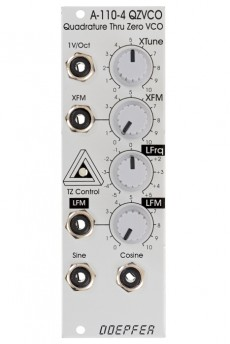A-110-4 is a so-called Thru Zero Quadrature VCO.

The term "quadrature" means in this connection that the oscillator outputs sine and cosine waveforms simultaneously. The term "Thru-Zero" means that even "negative" frequencies are generated. But this is a bit misleading term as negative frequencies do not really exist. "Negative" means in this connection simply that the sine/cosine waves will stop when the linear control voltage reaches 0V and continue with the opposite direction as the linear control voltage becomes negative and vice versa. The module has two control sections: linear and exponential. The exponential section consists of the XTune control, the 1V/Oct input and the XFM input with the corresponding attenuator XFM. The exponential control voltage is the sum of these three voltages. The linear section consists of the LTune control and the LFM input with the corresponding attenuator LFM. The linear control voltage is the sum of these two voltages.

The main advantage of the A-110-4 compared to other Thru Zero VCOs is that the design uses a sine/cosine core. The sine/cosine waves are not derived from other waveforms (e.g. sawtooth or triangle) by means of waveshaping. Rather the sine and cosine waves are the core of the VCO which results in very pure waves with a minimum of distortion and overtones.

Power consumption: 90mA at +12V and 30mA at -12V

Depth: 60mm

HP : 8

• A-110-4

140